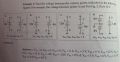# relative voltage point

#### Transatlantic

Joined Feb 6, 2014
44
These are some problems taken from the book 'Practical Electronics for Inventors (3rd Ed)'I'm wondering what I'm doing wrong as I get different answers for the ones marked in redb) Vac = 12v - 9v = 3v
Vbd = 0v - 0v = 0v
Vad = 12v - 0v = 12v
Vbc = 0v - 9v = -9v ????

c) Vac = 12v - 0v = 12v
Vbd = 0v - (-9v) = 9v ????
Vad = 12v - (-9v) = 21v ????

Vbc = 0v - 0v = 0v

#### MikeML

Joined Oct 2, 2009
5,444
Figure 2.13a. Note that there is absolutely no connection between the two batteries; they are effectively floating in space. Connecting a voltmeter that has a very high (say 100,000,000Ω) input resistance between node A and node C effectively connects those nodes together (albeit through a high resistance), so the voltage there is zero.Because B and D are open, there can be no current flow, so the voltage drop across the meter resistance is zero...

During this time, you could ask about the voltage between B and D, but the question is how do you propose to measure it? Another identical voltmeter? If so, then you have a voltage divider created by the two meters effectively in-series...

Last edited:

#### Jony130

Joined Feb 17, 2009
5,435
Well Practical Electronics for Inventors (3rd Ed) is a very bad book throw it away (too many errors).

As for 2.13b
Vac = 12V - 9V = 3V
Vbd = 0V - 0V = 0V
Vad = 12V - 0V = 12V
Vbc = 0V - 9V = - 9V

2.13c
Vac = 12V - 0V = 12V
Vbd = 0V - (-9V) = 9V
Vad = 12V - (-9V) = 21V
Vbc = 0V - 0V = 0V

2.13d
Vac = 3V - 0V = 3V
Vab = 3V - (-3V) = 6V
Vcd = 0V - 1.5V = -1.5V
Vad = 3V - 1.5V = 1.5V
Vbd = -3V - 1.5V = -4.5V

#### Transatlantic

Joined Feb 6, 2014
44
Interesting - so I guess no one has spotted the above erros then.

#### WBahn

Joined Mar 31, 2012
27,919
These are some problems taken from the book 'Practical Electronics for Inventors (3rd Ed)'

View attachment 88870

I'm wondering what I'm doing wrong as I get different answers for the ones marked in redb) Vac = 12v - 9v = 3v
Vbd = 0v - 0v = 0v
Vad = 12v - 0v = 12v
Vbc = 0v - 9v = -9v ????

c) Vac = 12v - 0v = 12v
Vbd = 0v - (-9v) = 9v ????
Vad = 12v - (-9v) = 21v ????

Vbc = 0v - 0v = 0v

#### WBahn

Joined Mar 31, 2012
27,919
Interesting - so I guess no one has spotted the above erros then.
Hard to tell. Most text books will have an errata sheet that you can get from the publisher (nowadays it's usually available from the website for the publisher, the author, or the textbook itself).

All texts have errors. I seldom use the term "always" but I'm "probably" safe in doing so this time. Check the errata. If it isn't listed, then that may indicate one of several things, none of which are particularly reassuring:

1) The book may be so seldom used that there aren't enough people seeing the error for there to be someone that reported it.
2) The author/publisher may have received reports but don't care about maintaining an up-to-date errata sheet.
3) There are just so many errors that it's a losing battle.
4) The book/edition hasn't been out long enough for the errors to get reported and the errata sheet to get updated.

The last one is the mildest and merely reflects the growing pains of a new text or new edition, but it's still no reassuring when you are working with a text that hasn't had time to get properly vetted. Of course, someone has to use it, so that's just one consideration.

The fact that you've got three errors out of seventeen trivially simple problems is NOT a good sign, though, whether they are on the errata sheet or not.

#### Jony130

Joined Feb 17, 2009
5,435

#### Transatlantic

Joined Feb 6, 2014
44
The fact that you've got three errors out of seventeen trivially simple problems is NOT a good sign, though, whether they are on the errata sheet or not.

5 errors#### WBahn

Joined Mar 31, 2012
27,919
5 errorsEven worse.

All of the answers for (a) are arguably incorrect. Since they are floating relative to each other, their potentials relative to each other are indeterminate, which is very different from 0V, which means that not only are they determinate, but that they are exactly the same. As MikeML alluded to, as soon as you go to measure one of the pairs you establish a reference, but that then changes all of the others. The problem ignores this, but doesn't say whether or not you have to make the measurements one at a time or whether you could use four voltmeters all at the same time. For the connected sources (i.e., Figures b, c, and d) this is a non-issue. But for (a) is it then end-all, be-all.